# Spectral Analysis of Linear Operators

## Spectral Analysis of Linear Operators

an extension, stemming from problems in mechanics, of the theory of eigenvalues and eigenvectors of matrices (that is, of linear transformations in finite-dimensional space) to the infinite-dimensional case (seeLINEAR OPERATOR and OPERATOR THEORY).

In vibration theory, the motion of a system with n degrees of freedom is studied in a neighborhood of a stable equilibrium position. This motion is described by a system of linear differential equations of the form + Ax = 0, where x is the n-dimensional vector of the deviations of the system’s generalized coordinates from their equilibrium values and A is a symmetric positive definite matrix. Such motion can be represented as a superposition of n harmonic motions, called normal modes, with angular frequencies equal to the square roots of the eigenvalues λk of A. The determination of the normal modes of the system reduces here to the finding of all the eigenvalues λk and eigenvectors xk of A.

The set of the eigenvalues of a matrix is called its spectrum. If A is a symmetric matrix, its spectrum consists of n real numbers λ1 . . . . λn, which are not necessarily distinct. By passing to a new coordinate system, the matrix can be reduced to diagonal form. In other words, the self-adjoint linear transformation A in n-dimensional space corresponding to the matrix admits of a special representation, called a spectral resolution, of the form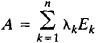where E1, . . . ,En are projection operators to the mutually perpendicular eigenvectors x1, . . . ,xn. On the other hand, if A is not a symmetric matrix or the associated non-self-adjoint linear transformation, then A in general has a spectrum consisting of complex numbers λ1, . . ., λn and can be reduced to a Jordan form that is more complicated than the diagonal form (see JORDAN MATRIX) and that corresponds to a more complicated representation of the linear transformation A than the ordinary spectral resolution described above.

In studying vibrations near an equilibrium state of systems with an infinite number of degrees of freedom—for example, the vibrations of a homogeneous or nonhomogeneous string—the problem of determining the eigenvalues and eigenvectors of a linear transformation in finite-dimensional space must be extended to some class of linear transformations, that is, linear operators, in infinite-dimensional space. In many cases—in particular, in the case of a vibrating string, the corresponding operator can be written in the form of an integral operator A acting in the space of functions f(x), so thatwhere K(x, y) is a continuous function of two variables that is defined in the square ax and yb and satisfies the symmetry condition K(x, y) = K(y, x). In these cases, the operator A always has a complete system of pairwise orthogonal eigenfunctions φk, to which there corresponds a countable sequence of real eigenvalues λk that collectively form the spectrum of A. If the functions on which A operates are regarded as vectors in a Hilbert space, then, as in the case of a finite-dimensional self-adjoint transformation, the action of A reduces to stretching the space along the system of mutually orthogonal axes φk by λk. (When λk < 0, we mean a stretching by ǀλkǀ followed by a reflection.) A here has a spectral resolution of the form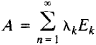where the Ek are projection operators to the φk.

Spectral analysis was first developed for integral operators with a symmetric kernel K(x, y) that is defined and continuous in some bounded region. Subsequently, within the framework of general operator theory, spectral analysis was extended to many other types of linear operators (for example, integral operators whose kernel has a singularity or is defined in an unbounded region and differential operators in spaces of functions of one or more variables) and to abstractly defined linear operators in infinite-dimensional linear spaces. This extension, however, substantially complicated spectral analysis, since for many linear operators eigenvalues and eigenfunctions, in the ordinary sense, do not exist. In the general case, therefore, the spectrum must be defined not as the set of eigenvalues of A but as the set of numbers X such that the operator (A – λE)-1, where E is the identity operator, does not exist, is defined only on a nondense set, or is unbounded. All the eigenvalues of the operator belong to its spectrum and collectively form its point spectrum; the remainder of the spectrum is often called the continuous spectrum of the operator. The continuous spectrum, however, is sometimes understood to mean only the set of λ such that the operator (A - λE)–1 is defined on a dense set of elements of the space but is unbounded ; points of the spectrum that are in neither the point nor the continuous spectrum, are then called the residual spectrum.

The most thoroughly developed areas of spectral analysis deal with self-adjoint linear operators in Hubert space, which are generalizations of symmetric matrices, and with unitary operators in Hubert space, which are generalizations of unitary matrices. A self-adjoint operator A in Hubert space always has a purely real spectrum (point, continuous, or mixed) and admits of a spectral resolution of the form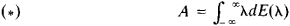Here, E(λ) is called the resolution of unity corresponding to A and is a family of projection operators satisfying special conditions. In this case, the points of the spectrum are points at which the operator function E(λ) increases. In the case of a purely point spectrum, all the points are jump discontinuities, so that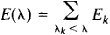and the spectral resolution (*) reduces to the expansion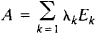A unitary operator in Hubert space has its spectrum on the circle ǀλǀ = 1 and admits of a spectral resolution related in form to (*) but with integration from –∞ to ∞ replaced by integration along the circle. The special class of normal operators in Hubert space is also studied. These operators can be represented in a form similar to (*), but the integration on the right side is extended to the more general set of points λ in the complex plane that now constitute the spectrum of A.

Much work has been done on the spectral analysis of nonnor-mal and non-self-adjoint linear operators that generalize non-symmetric matrices. Studies have been made in this area by many mathematicians, including T. Carleman of Sweden, B. Szőkefalvi-Nagy of Hungary, M. V. Keldysh and M. G. Krein of the USSR, and G. Birkhoff and N. Dunford of the USA. Nonetheless, the theory of such spectral analysis is still far from being complete.

The spectral analysis of linear operators has a number of important applications in classical mechanics (particularly in vibration theory), electrodynamics, quantum mechanics, the theory of stochastic processes, the theory of differential and integral equations, and other areas of mathematics and mathematical physics.

### REFERENCES

Courant, R., and D. Hubert. Melody matematicheskoifiziki, 3rd ed., vol. 1. Moscow-Leningrad, 1951. (Translated from German.)
Akhiezer, N. I., and I. M. Glazman. Teoriia lineinykh operatorov v gil’bertovom prostranstve, 2nd ed. Moscow, 1966.
Plesner, A. I. Spektral’naia teoriia lineinykh operatorov. Moscow, 1965.
Riesz, F., and B. Szőkefalvi-Nagy. Lektsiipo funktsial’nomy analizu. Moscow, 1954. (Translated from French.)
Szőkefalvi-Nagy, B., and C. Foias. Garmonicheskii analiz operatorov v gil’bertovom prostranstve. Moscow, 1970. (Translated from French.)
Dunford, N., and J. T. Schwartz. Lineinye operatory, parts 2–3. Moscow, 1966–74. (Translated from English.)
Keldysh, M. V., and V. B. Lidskii. “Voprosy spektral’noi teorii nesa-mosopriazhennykh operatorov.” In Tr. 4-go Vsesoiuznogo male-maticheskogo s”ezda, vol. 1. Leningrad, 1963. Pages 101–20.
Site: Follow: Share:
Open / Close